### 14.6.3.5 A bang-bang approach for time optimality

The dynamic programming approach is already very efficient because the search is confined to two dimensions. Nevertheless, trajectories that are time optimal can be computed even more efficiently if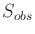has some special structure. The idea is to find an alternating sequence between two motion primitives: one of maximum acceleration and one of maximum deceleration. This kind of switching between extreme opposites is often called bang-bang control and arises often in the development of time-optimal control laws (look ahead to Example 15.4). The method explained here was introduced in [121,879]. One drawback of obtaining time-optimal trajectories is that they cannot be tracked (the fourth module from Section 14.6.1) if errors occur because the solutions travel on the boundary of the reachable set.

The approach was developed for robot arms, as considered in Example 14.7. Suppose thatis a single connected component that is bounded above by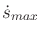, and on the sides it is bounded by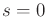and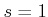. It is assumed that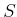arises only due to the vanishing of the interval of allowable values for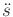(in this case,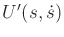becomes empty). It is also assumed that the lower boundary ofcan be expressed as a differentiable function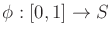, called the limit curve, which yields the maximum speed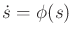for every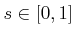. The method is extended to handle multiple obstacles in , but this case is not considered here. Assume also that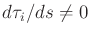for every; the case of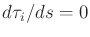can also be handled in the method .

Let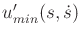and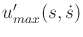denote the smallest and largest possible accelerations, respectively, from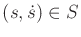. If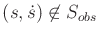, then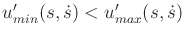. At the limit curve,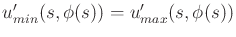. Applying the only feasible action in this case generates a velocity that is tangent to the limit curve. This is called a tangent point,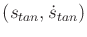, to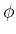. Inside of, no accelerations are possible.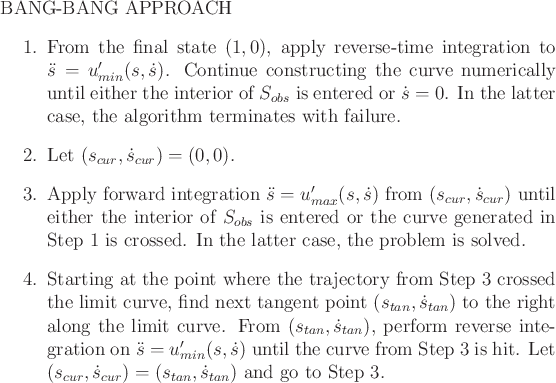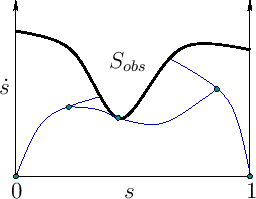The bang-bang approach is described in Figure 14.28, and a graphical illustration appears in Figure 14.29. Assume that the initial and goal phases are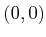and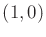, respectively. Step 1 essentially enlarges the goal by constructing a maximum-deceleration curve that terminates at. A trajectory that contacts this curve can optimally reachby switching to maximum deceleration. Steps 3 and 4 construct a maximum-acceleration curve followed by a maximum-deceleration curve. The acceleration curve runs until it pierces the limit curve. This constraint violation must be avoided. Therefore, a deceleration must be determined that departs earlier from the acceleration curve and just barely misses entering the interior of. This curve must become tangent to the limit curve; therefore, a search is made along the limit curve for the next possible tangent point. From there, reverse-time integration is used in Step 4 to generate a deceleration curve that contacts the acceleration curve. A portion of the solution has now been obtained in which an acceleration is followed by a deceleration that arrives at a tangent point of. It is possible that Step 4 is not reached because the curve that connects to the goal is contacted. Starting from the tangent point, Steps 3 and 4 are repeated until the goal curve is contacted.

Steven M LaValle 2020-08-14